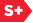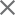### Proceedings Paper

PHLFT5: a practical and improved version of polyharmonic local Fourier transform
Author(s): Jucheng Zhao; Naoki Saito; Katsu Yamatani
Format Member Price Non-Member Price
PDF \$17.00 \$21.00

Paper Abstract

We introduce a practical and improved version of the Polyharmonic Local Fourier transform (PHLFT) called PHLFT5. After partitioning an input image into a set of rectangular blocks, the original PHLFT decomposes each block into the polyharmonic component and the residual. The polyharmonic component solves the polyharmonic equation with the boundary condition that matches the values and normal derivatives of first order up to higher order of the solution along the block boundary with those of the original image block. Thanks to this boundary condition, the residual component can be expanded into a complex Fourier series without facing the Gibbs phenomenon and its Fourier coefficients decay faster than those of the original block. Due to the difficulty of estimating the higher order normal derivatives, however, only the harmonic case (i.e., Laplace's equation) has been implemented to date. In that case, the Fourier coefficients of the residual decay as O (||k||-2) where k is the frequency index vector. Unlike the original version, PHLFT5 only imposes the boundary values and the first order normal derivatives as the boundary condition, which can be estimated using our robust algorithm. We then derive a fast algorithm to compute a fifth degree polyharmonic function that satisfies such boundary condition. The Fourier coefficients of the residual now decay as O (||k||-3). We shall also show our preliminary numerical experiments that demonstrates the superiority of PHLFT5 over the original PHLFT of harmonic case in terms of the decay rate of the residual and interpretability of oriented textures.

Paper Details

Date Published: 17 September 2005
PDF: 12 pages
Proc. SPIE 5914, Wavelets XI, 59141N (17 September 2005); doi: 10.1117/12.618584
Show Author Affiliations
Jucheng Zhao, Univ. of California, Davis (United States)
Naoki Saito, Univ. of California, Davis (United States)
Katsu Yamatani, Shizuoka Univ. (Japan)

Published in SPIE Proceedings Vol. 5914:
Wavelets XI
Manos Papadakis; Andrew F. Laine; Michael A. Unser, Editor(s)